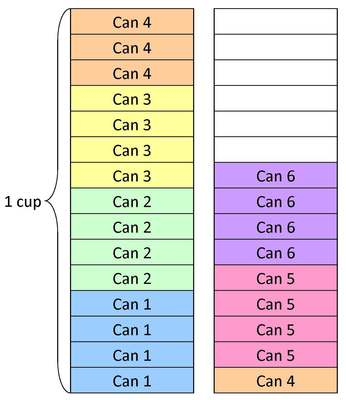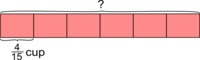# Sugar in six cans of soda

Alignments to Content Standards: 4.NF.B.4.c

For a certain brand of orange soda, each can contains $\frac{4}{15}$ cup of sugar.

1. How many cups of sugar are there in six cans of this orange soda?
2. Draw a picture representing the answer to (a).

## IM Commentary

This task provides a familiar context allowing students to visualize multiplication of a fraction by a whole number. This task could form part of a very rich activity which includes studying soda can labels. Unfortunately, the information about sugar content is usually given in grams and converting grams of sugar to cups of sugar is not in the fourth grade standards. Nonetheless work on this problem can pave the way to a richer discussion of this conversion when it is appropriate in the middle school grades.

Notice in the picture for part (b) that the second cup of sugar has been cut into fifteen equal pieces even though only nine of these are filled up by the sugar from the soda. It is important to show this division of the cup into fifteen equal pieces, even though not all of them are filled with sugar, because this tells us that the equal amounts of sugar represented in the picture are each fifteenths of a cup.

This task is appropriate for instruction or assessment.

The Standards for Mathematical Practice focus on the nature of the learning experiences by attending to the thinking processes and habits of mind that students need to develop in order to attain a deep and flexible understanding of mathematics. Certain tasks lend themselves to the demonstration of specific practices by students. The practices that are observable during exploration of a task depend on how instruction unfolds in the classroom. While it is possible that tasks may be connected to several practices, only one practice connection will be discussed in depth. Possible secondary practice connections may be discussed but not in the same degree of detail.

This particular task helps illustrate Mathematical Practice Standard 4, Model with mathematics. Students apply the mathematics they know to solve problems arising in everyday life. For this problem, fourth graders might apply their understanding of multiplication as repeated addition and multiply 4/15 cups of sugar 6 times, draw a picture depicting the sugar in 6 cans of soda, or create a bar diagram with each of the six sections representing 4/15 of sugar. As students think about the situation in real life, they have to select the mathematical thinking that will help them solve the problem.  This selection of a mathematical model allows students to think about which mathematics might be useful in the real life situation that they face.  Students identify the mathematical elements of the situation and decide which solution pathway is best for them to follow.

## Solutions

Solution: 1

1. There are six cans of soda and each one contains $\frac{4}{15}$ cup of sugar. In the six cans of soda, there are $6$ groups of $\frac{4}{15}$ cup of sugar. To find out how much sugar there is in the six cans, this is a multiplication problem: 6 groups of $\frac{4}{15}$ cups of sugar is $$6 \times \frac{4}{15}$$ cups of sugar. To find the solution,

\begin{align} 6 \times \frac{4}{15} &= \frac{6 \times 4}{15} \\ &= \frac{24}{15} \\ &= 1\frac{9}{15} . \end{align}
So there are $\frac{24}{15} = 1\frac{9}{15}$ cups of sugar in the six cans of orange soda. To see the reasoning behind the first equation in the sequence above, 6 groups of $\frac{4}{15}$ cups is the same as adding $\frac{4}{15}$ to itself six times: so the denominator of the result will be 15 (all quantities being added are fifteenths of a cup) while the numerator is a sum of six fours or $6 \times 4$.

2. Below is a picture showing the sugar in the 6 cans of soda. There are two cups, each of which has been divided into 15 equal pieces. Each can of soda contains $\frac{4}{15}$ cup of sugar: this is why each can is listed four times, once for each $\frac{1}{15}$ of a cup of sugar contained in that can. We see from the picture that the six cans of soda contain one full cup of sugar plus part of another. Counting the parts in the second cup, we see that $\frac{9}{15}$ of the second cup has been filled with the sugar from the soda. So, in total, the six cans of orange soda contain $1 \frac{9}{15}$ or $\frac{24}{15}$ cups of sugar.Solution: Using a bar diagram1 can: $\frac{4}{15}$ cup

6 cans: $6 \times \frac{4}{15} = \frac{6\times4}{15} = \frac{24}{15} = 1 \frac{9}{15} = 1 \frac{3}{5}$ cups

There would be $1 \frac{3}{5}$ cups of sugar in 6 cans of soda.